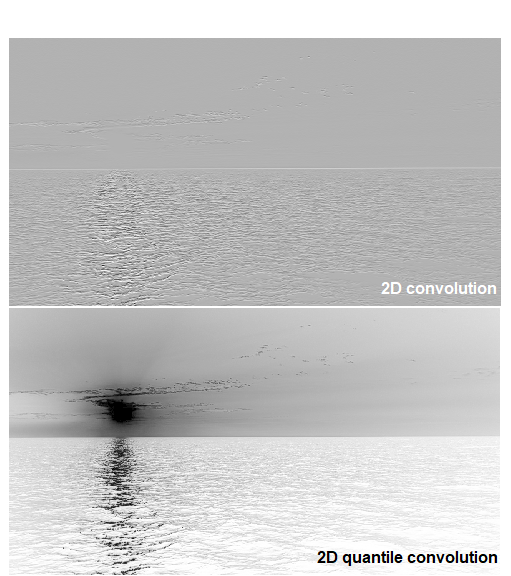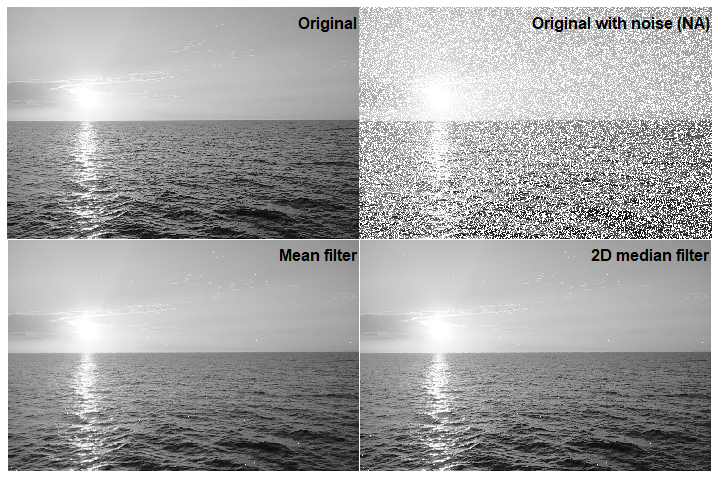# imagine

IMAGing engINE, Tools for Application of Image Filters to Data Matrices

This package was built up to take numeric data matrices and apply image-filtering algoriths.

Algoriths used by imagine include median-filter and 2D-convolution based algoriths performed on Rcpp (C++) in order to speed up the application of this filters to large numeric matrices.

## Installation

For installing imagine, as follows:

install.packages("imagine")

## Engines

imagine performs algorithms (called ‘engines’) written in C++ using Rcpp and RcppArmadillo, ensuring the faster application of filters. At version 2.0.0, imagine includes four main engines, described as follows:

• Engine 1: Basic 2D-convolution algorithm. It multiplies kernel by the neighborhood of each cell and then it sums all values.
• Engine 2: Make the same of Engine 1 but, at the end, it returns the position indicated by the parameter probs.
• Engine 3: It uses the radius argument to extract the values of squared neighborhood ($$radius \times radius$$) and calculates the mean.
• Engine 4: It uses the radius argument to extract the values of squared neighborhood ($$radius \times radius$$) and returns the position indicated by the parameter probs

Since version 2.0.0, radius could accept 2 values to define the number of rows and columns respectively of the window.

## Main functions

There are 5 main functions and 2 wrappers:

### Convolution functions

# Build kernels
# Kernel 1: For bottom edge recognition
kernel1 <- matrix(c(-1, -2, -1,
0,  0,  0,
1,  2,  1),
nrow = 3)

# Kernel 2: Diagonal weighting
kernel2 <- matrix(c(-2, 0, 0,
0, 1, 0,
0, 0, 2),
nrow = 3)

# Apply filters
convolutionExample  <- convolution2D(X = wbImage, kernel = kernel1)
convQuantileExample <- convolutionQuantile(X = wbImage, kernel = kernel2, probs = 0.1)

In order to compare results, we will plot both data (original and filtered) using image function, as shows in figures 1 and 2.

Original vs filtered outputsFigure 1: 2D vs 2D quantile convolutions

### Median-filter asociated functions

# Add some noise (NA) to the image (matrix)
set.seed(7)
naIndex <- sample(x       = seq(prod(dim(myMatrix))),
size    = as.integer(0.4*prod(dim(myMatrix))),
replace = FALSE)
myMatrix[naIndex] <- NA

# Build kernel

# Apply filters
medianfilterExample   <- medianFilter(X = myMatrix, radius = radius)

Now, we will plot both data (original and filtered) using image function, as shows in figures 1 and 2.

Original and FilteredFigure 2: Basic filters comparison

## Kernel application

In the field of image processing, one of the tools most commonly used are the convolutions, which consist of operations between two arrays: The array of image data (as a big matrix) and kernels (as small matrices) which weighs each pixel values by the values of its corresponding neighborhood. Different kernels produce different effects, for instance: blur, shifted images (right, left, up or down), sharpening, etc. The users must be cautious with the size of the kernel because the larger the radius, the more pixels remain unanalyzed at the edges.

Besides, every function of imagine allows the recursive running of a filter by the using of times argument.

medianFilter(X = wbImage, radius = 5, times = 50)